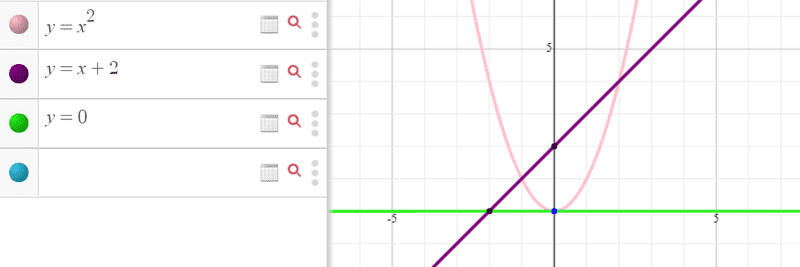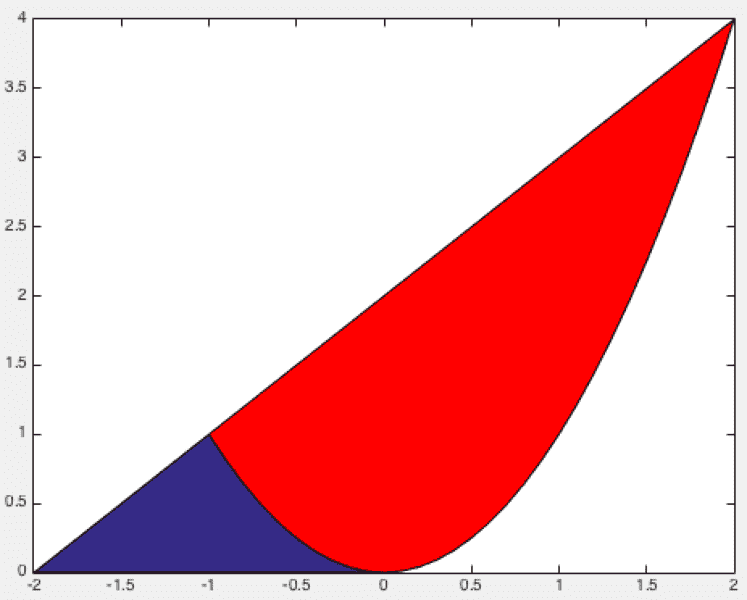# Find the volume using the shell method

## Homework Statement

Use the shell method to find the volume of the solid generated by revolving the region bounded by the line y = x + 2 and the parabola y=x^2 about the x- axis.

-

## The Attempt at a Solution

1) x = √ (y)
2) x= y -2
V = ∫ (2 π ) ( y ) ( √ (y) - y + 2 ) from 0 to 4
= 46.9
(( The correct answer is (72 π / 5 ) ))

Last edited:

Orodruin
Staff Emeritus
Homework Helper
Gold Member
Please describe what you are doing rather than just writing down formulas. It will help us in assessing where your thinking goes wrong.

Please describe what you are doing rather than just writing down formulas. It will help us in assessing where your thinking goes wrong.
( 1 ) y = x^2 ---> x = √ y
( 2 ) y = x + 2 ----> x = y - 2
the shell thickness variable is y , so the limits of the integration for the shell formula method are a = 0 and b = 4 (along the y-axis).
shell height = ( 1 ) - ( 2 ) = √ y - y - 2
V = ∫ 2 π ( shell radius ) ( shell height ) from a to b
= ∫ 2 π ( y )( √ y - y - 2) from 0 to 4
= 46.9

Orodruin
Staff Emeritus
Homework Helper
Gold Member
You seem to be assuming that the lower bound on ##x## is always given by ##x = y - 2##. I suggest that you draw the functions ##y = x+2## and ##y = x^2## to see if this is really the case. (For example, why did you choose to integrate from ##y = 0##? The function ##y = x+2 \geq 1## in your entire interval for ##x##.)

You seem to be assuming that the lower bound on ##x## is always given by ##x = y - 2##. I suggest that you draw the functions ##y = x+2## and ##y = x^2## to see if this is really the case. (For example, why did you choose to integrate from ##y = 0##? The function ##y = x+2 \geq 1## in your entire interval for ##x##.)#### Attachments

Last edited:
Mark44
Mentor
@Fatima Hasan, the region being revolved is bounded by the parabola and the line. Part of this region is in the 2nd quadrant. Your graph shows the parabola starting from the origin -- the parabola intersects the line at a second point that isn't shown on your graph.

Also, if you use shells to calculate the volume, you need two integrals, not one, since the left endpoint of your typical area element changes. In one integral, the endpoints of the area element are on the left and right branches of the parabola. In the other integral, the endpoints of the area element are on the line and the right branch of the parabola. As a result, the value you are getting is slightly too large -- you're including a part of the region that should be excluded.

•Fatima Hasan
Orodruin
Staff Emeritus
Homework Helper
Gold Member
Just to be extra specific:You are computing the volume resulting from revolving both the red and the blue area around the x-axis. The question asks you to find the area resulting from revolving just the red area. (Click for larger image.)

To be honest, this question is easier to solve using the disc method as you only need one case and can integrate from ##x = -1## to ##x = 2##.

Edit: You can solve this either by splitting the red area in separate integrations or by subtracting the volume of revolution of the blue area from your first result.

#### Attachments

•Fatima Hasan
LCKurtz